home |  avr microcontroller & DIY electronics |  toolbox |  science club |  tuxtalk |  photos |  e-cards |  online-shop# A short explanation on how to use the Chinese abacus (suanpan)

Click on the beads to move them.

## The value of the beads and how to read numbers from the abacus

Look at the abacus and play around with it. It is a fully working abacus so you can try out all the examples we will be looking at!
Okay, look at the abacus. It has several colums with 5 beads on each column of the lower deck and 2 beads on each colum of the upper deck.
In its start position or when it shows 0, the beads in the lower deck are all moved down while the beads in the upper deck are all moved up.

The five beads of each colum of the lower deck together have the same potential value as one of the 2 beads of each colum of the corresponding upper deck.
We start with the 5 beads in the lower deck of the column the farthest to the right. Here each bead has the potential value 1. The 2 beads in the upper deck of that column each have the potential value of 5.
The 5 beads in the lower deck of the second column from the right each have the potential value 10, the 2 beads in the upper deck of the second column from the right each have the potential value 50, the 5 beads in the lower deck of the third column from the right each have the potential value 100 and so on.
And as soon as you move a bead you actually give it its value.

So number 3 looks like this: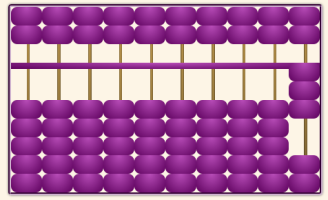The next picture shows the number 8: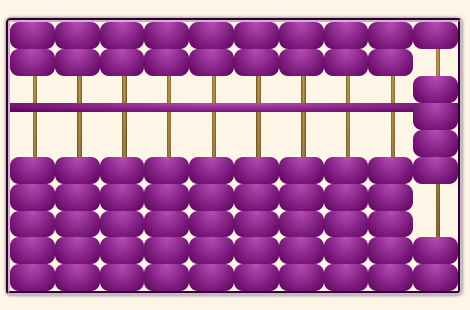And finally number 258: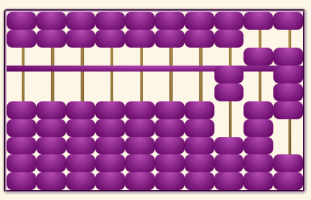Let's look at a very simple addition to see how it works on the abacus: Let's add 4 +3.
After bringing the abacus in its starting position you begin with displaying the first number, the 4 in our case. For this you move 4 beads from the column of the lower deck from the farthest right up. Now the abacus shows the 4.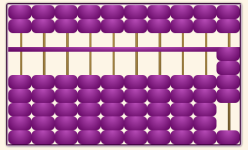Then you need to add three more beads. After moving one more bead up there are no more beads left to move up for the two that still need to be moved upward. So we split the 3 in 1+2 in our head and change the 5 moved-up beads with one bead from the upper deck from the same column. We move that bead and the other 5 down. Now we have enough free beads again to move the remaining 2 beads up. That's it. We are done and you can see that 4 + 3 makes 7.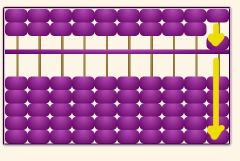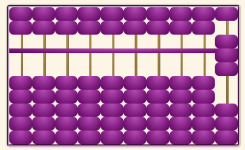Let's take another example: 63 + 39.
Again we put all the beads in their starting position. Then we first display the 63 which contains three ones and 6 tens. For the three ones we simply move the three beads in the first column of the lower deck upwards. The 6 is bigger than 5 so we split it into 5 + 1. In the second column we move one bead from the upper deck down and one bead from the lower deck up.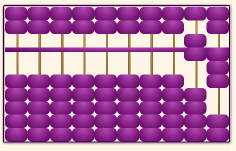Now we still need to add the 3 tens to our abacus. But before we should change the 2 value5 beads into one of value 10, that is one bead of the lower deck from the second column from the right.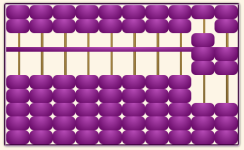To add the three tens we need to look at the lower deck of the second column from the right. There are exactly 3 left! So we move them up. As all are upwards we need to exchange them for one bead of the upper deck from the same column.
But now the two beads of the upper deck are both down. So we need to change them too into one bead of value100, that is one bead from the lower deck of the third column from the right.
And now we are done and you can read from the abacus that 63 + 39 is 102.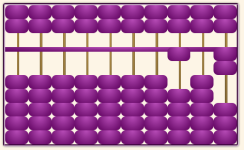## Subtraction

For understanding how subtraction is done with the abacus let's revisit our first example, just make it a subtraction now: 7-4.
First you have to display the 7 on the abacus. We know that we need to move one bead from the upper deck down and two beads from the lower deck of the column farthest to the right up. Now we have the 7.Now we need to remove 4. On the lower deck we only have two beads that we can remove which is not enough. But we first remove them knowing that we still have to remove 2 more.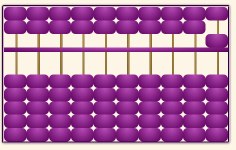Now with the two beads moved down we can dissolve the value5 bead from the upper deck into 5 value1 beads from the lower deck which gives us again more beads to remove.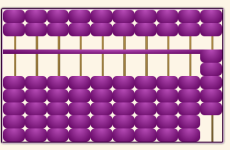We can now easily remove the 2 beads and we are done. Now we can see that 7-4 makes 3.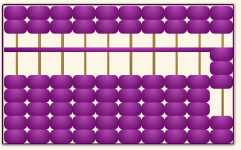The important idea for subtraction is that you always need to dissolve a higher value bead into lower value beads as often as necessary to remove all the beads that need to be removed. It is just the reverse from addition where we always exchanged the lower valued beads for a higher valued one.
To see this idea in action again we look at two more examples. First we calculate 20-8. Again clear your abacus so that it is back in the start position. Then feed the 20 into it. 20 is 0 ones and 2 tens. So we leave the first column from the right untouched and just move two beads from the lower deck of the second column from the right up. Now we have 20.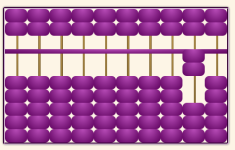If we now want to remove 8 we can't do it directly but first need to dissolve one of the value10 beads into a value5 and 5 value1 beads.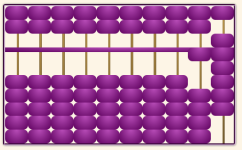After having done this removing 8 is easy. We simply move the value5 bead back up and move 3 of the value1 beads down. Now we are done and can read our result which is 12.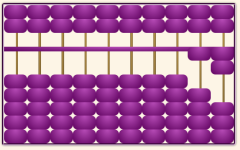Next let's calculate 1000-16. Again clear the abacus and bring it into its start position. Then feed the 1000 into the abacus. For this we move one bead of the lower deck from the fourth column from the right up.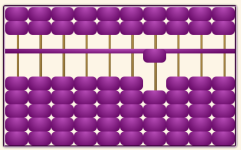Again we cannot remove 16 directly but first need to disolve the 1000 into smaller parts. Imagine that you had a 1000\$ bill and would change it into smaller bills so that you could give someone 16\$. So first we move the the bead from the fourth column back down and then from the third column we move one bead from the upper deck down and all 5 beads from the lower deck up.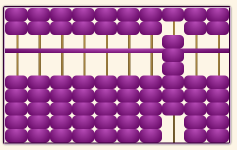Having done this we still can't subtract 16 but need to disolve the beads further. So we move one bead from the lower deck of the third column down and from the second column we move one bead from the upper deck down and all 5 beads from the lower deck up.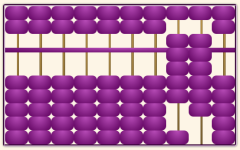And we are still not done. We still need to repeat this for column 2 again. Move one bead from column 2 down and instead you move one bead from the upper deck down and all five beads from the lower bead up.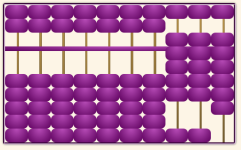Now we are finally able to subtract our 16. First we remove the 6 from the first column. For this we move the one value5 bead up and one value1 bead down.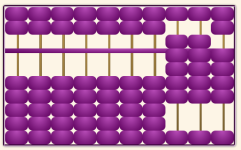So we only need to subtract the 10 which is done simply by moving one bead from the lower deck of the second column down. Now we are done and can read the result from the abacus: In the third column we have 4 beads in the lower deck and 1 in the upper deck which gives us 9. In the second column we habe 3 beads in the lower deck and one in the upper which makes 8 and in the first column we just have the four beads from the lower deck so we have 4 which together gives us the number 984, so now we know that 1000-16=984.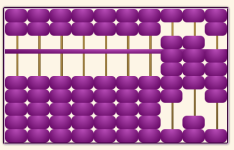## Simple multiplication and division: if you don't know your multiplication and division tables

If you are weak with your multiplication and division of two one digit numbers you can use your abacus as well. Let's take 6*4 as an example. To calculate this you would simply feed 6 into the abacus 4 times.
If you wanted to calculate 24:8 you would feed the 24 into the abacus first. Then you remove group of 8s until none is left. To help you not to lose track of your count you move one bead from the first column of the left up for each 8 removed.

To do multiplication and division with bigger numbers is harder. But let's at least have a look at how multiplication can be done.

## Multiplication

Let's take 67 * 8 as our example. Again first clear the abacus and bring the beads into its start position. Then to remember the two numbers that need to be multiplied you feed them into the abacus on the left (not the right). First feed the six into the last column from the right (or the first column from the left), then the 7 in the column next to it. Now leave a vacant column between the numbers and then on the fourth column to the left feed the 8 into our abacus.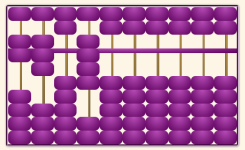Now we start by multiplying 7 and 8. This makes 56. So we feed the 56 into the abacus. The 6 goes into the first column from the right and the 5 into the second.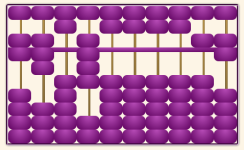After this is done we calculate 6*8 which makes 48. As what we really wanted to caluclate was 60*8 which makes 480 the 8 goes into the second column and the 4 in the third.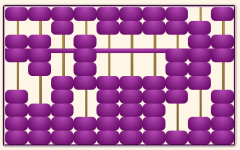Now we just need some rearraging to do: the two upper beads from the second column are exchanged with one lower bead from the third column. As now this column has all 5 lower beads up we can exchange them for one upper bead in that column. And now you can easily read the answer 536 from the abacus.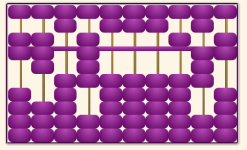Let's look at one more example: 75 * 96. Again after clearing the abacus and bringing it into its start position you feed the two numbers into the abacus. From the left it's the 7 in the first column, the 5 in the second, the third column remains untouched, then the 9 goes in the fourth column and the 6 in the fifth.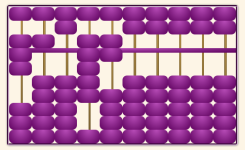Now we start calculating. First we calculate 5*6 which makes 30. So we feed that number in, 0 in the first column from the right and 3 in the second column from the right.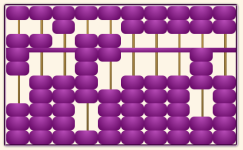Next it's 7*6=42, as this is really 70*6=420 the 2 goes in the second column and the 4 in the third (again from the right). As now all lower beads in the second column are up we rearrange them by putting all lower beads down and one of the upper beads down instead.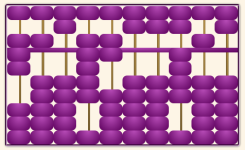As the 6 now has done its part we can remove it from the fifth column from the left. That way we don't get confused about what we have already calculated and if we had bigger numbers we also got more space for our calculations.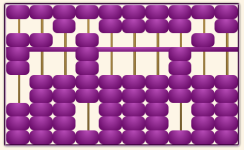Next is 9*5=45 and again as it's really 90*5=450 the five goes in the second column from the right and the 4 in the third. After rearranging we get: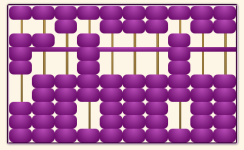So now we only need to calculate 9*7=63 (or 90*70=6300) and plug in the 3 in the third and the 6 into the fourth column from the right and after rearranging we have the answer 7200.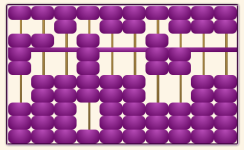If you want to know about the Chinese abacus I recommend reading the book "The abacus" by Jesse Dilson. It's a very nice book that even comes with a little wooden abacus and everything is explained nicely in there. You get lots of background information aabout the abacus and will even be able to perform divisions after reading the book.

And now have lots of fun playing around with the abacus! :)